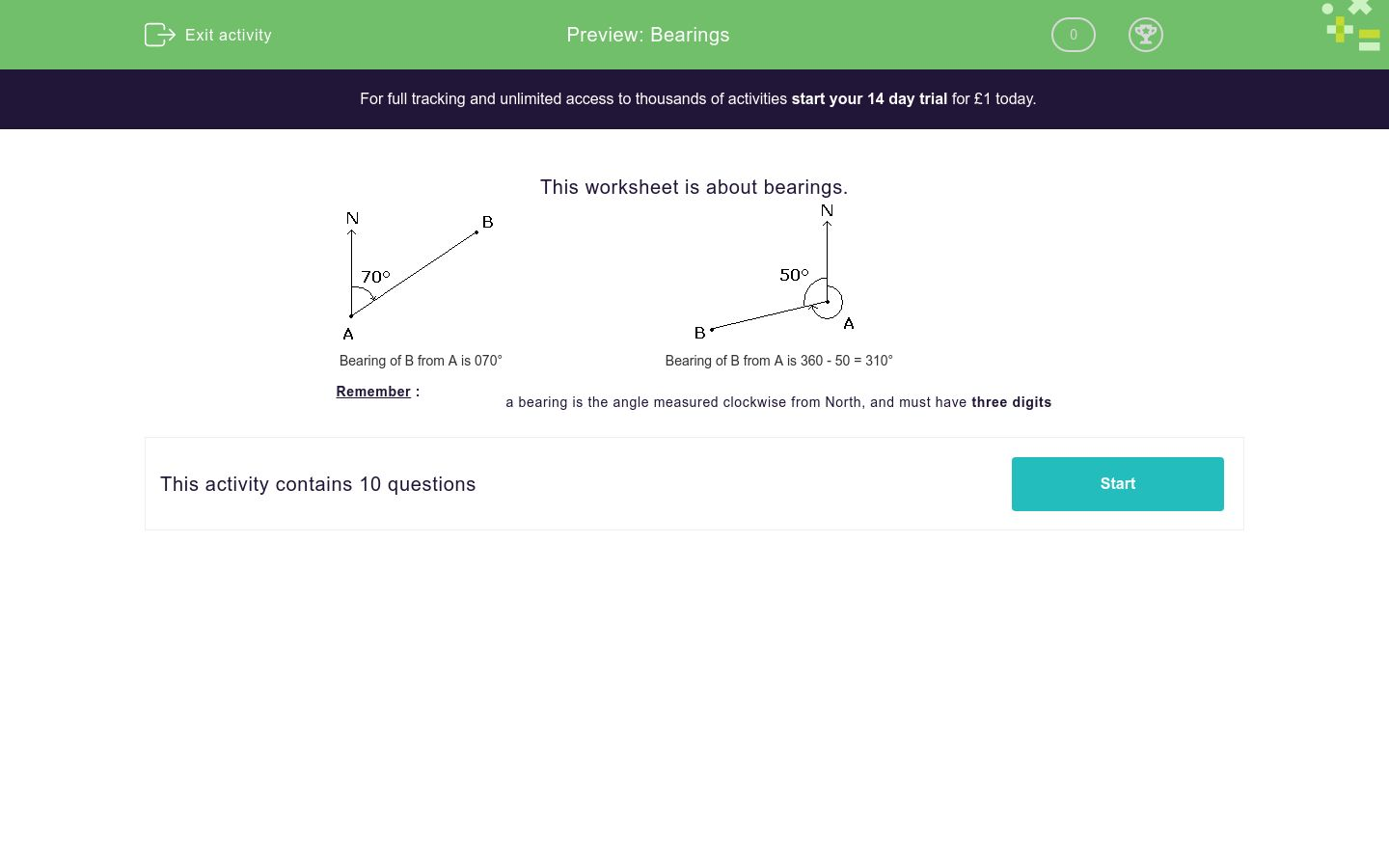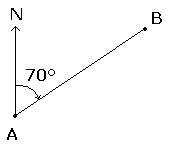# Bearings

In this workshsheet, students calculate bearings from the given angles.Key stage:  KS 4

Curriculum topic:  Geometry and Measures

Curriculum subtopic:  Interpret and Use Bearings

Difficulty level:### QUESTION 1 of 10

This worksheet is about bearings.Bearing of B from A is 070° Bearing of B from A is 360 - 50 = 310° Remember : a bearing is the angle measured clockwise from North, and must have three digits

In the following diagram, work out the bearing of B from A .x = 40°
Bearing of B from A =
 ?

Just write the number in the text box below.

In the following diagram, work out the bearing of B from A .x = 74°
Bearing of B from A =
 ?

Just write the number in the text box below.

In the following diagram, work out the bearing of B from A .y = 156°
Bearing of B from A =
 ?

Just write the number in the text box below.

In the following diagram, work out the bearing of B from A .x = 5°
Bearing of B from A =
 ?

Just write the number in the text box below.

In the following diagram, work out the bearing of B from A .x = 14°
Bearing of B from A =
 ?

Just write the number in the text box below.

In the following diagram, work out the bearing of B from A .x = 68°
Bearing of B from A =
 ?

Just write the number in the text box below.

In the following diagram, work out the bearing of B from A .x = 6°
Bearing of B from A =
 ?

Just write the number in the text box below.

In the following diagram, work out the bearing of B from A .y = 112°
Bearing of B from A =
 ?

Just write the number in the text box below.

In the following diagram, work out the bearing of B from A .x = 6°
Bearing of B from A =
 ?

Just write the number in the text box below.

In the following diagram, work out the bearing of B from A .x = 8°
Bearing of B from A =
 ?

Just write the number in the text box below.

• Question 1

In the following diagram, work out the bearing of B from A .x = 40°
Bearing of B from A =
 ?

Just write the number in the text box below.

CORRECT ANSWER
140
EDDIE SAYS
180 - 40 = 140
• Question 2

In the following diagram, work out the bearing of B from A .x = 74°
Bearing of B from A =
 ?

Just write the number in the text box below.

CORRECT ANSWER
074
EDDIE SAYS
Three digits needed, so not just 74.
• Question 3

In the following diagram, work out the bearing of B from A .y = 156°
Bearing of B from A =
 ?

Just write the number in the text box below.

CORRECT ANSWER
204
EDDIE SAYS
360 - 156 = 204.
• Question 4

In the following diagram, work out the bearing of B from A .x = 5°
Bearing of B from A =
 ?

Just write the number in the text box below.

CORRECT ANSWER
175
EDDIE SAYS
180 - 5 = 175
• Question 5

In the following diagram, work out the bearing of B from A .x = 14°
Bearing of B from A =
 ?

Just write the number in the text box below.

CORRECT ANSWER
014
EDDIE SAYS
Three digits needed, so not just 14.
• Question 6

In the following diagram, work out the bearing of B from A .x = 68°
Bearing of B from A =
 ?

Just write the number in the text box below.

CORRECT ANSWER
112
EDDIE SAYS
180 - 168 = 112
• Question 7

In the following diagram, work out the bearing of B from A .x = 6°
Bearing of B from A =
 ?

Just write the number in the text box below.

CORRECT ANSWER
006
EDDIE SAYS
Three digits needed, so not just 6.
• Question 8

In the following diagram, work out the bearing of B from A .y = 112°
Bearing of B from A =
 ?

Just write the number in the text box below.

CORRECT ANSWER
248
EDDIE SAYS
360 - 112 = 248
• Question 9

In the following diagram, work out the bearing of B from A .x = 6°
Bearing of B from A =
 ?

Just write the number in the text box below.

CORRECT ANSWER
174
EDDIE SAYS
180 - 6 = 174
• Question 10

In the following diagram, work out the bearing of B from A .x = 8°
Bearing of B from A =
 ?

Just write the number in the text box below.

CORRECT ANSWER
172
EDDIE SAYS
180 - 8 = 172
---- OR ----

Sign up for a £1 trial so you can track and measure your child's progress on this activity.

### What is EdPlace?

We're your National Curriculum aligned online education content provider helping each child succeed in English, maths and science from year 1 to GCSE. With an EdPlace account you’ll be able to track and measure progress, helping each child achieve their best. We build confidence and attainment by personalising each child’s learning at a level that suits them.

Get started#### Similar activities you may like

Start your £1 trial today.
Subscribe from £10/month.